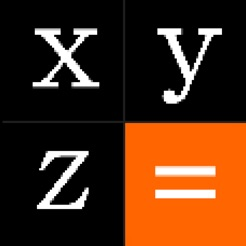## iPhone Screenshots

•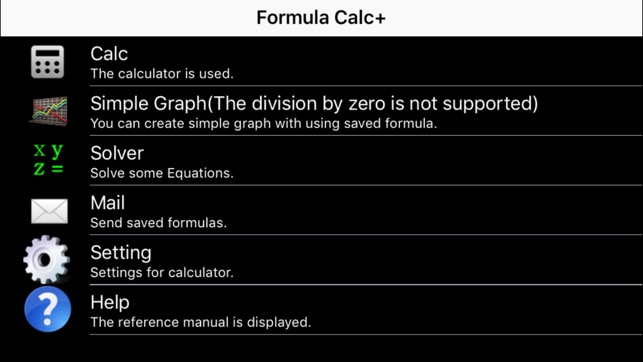•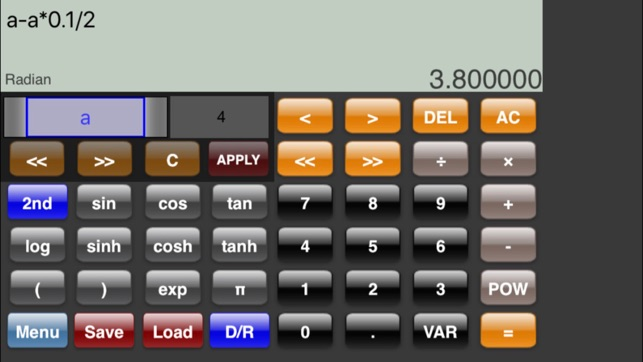••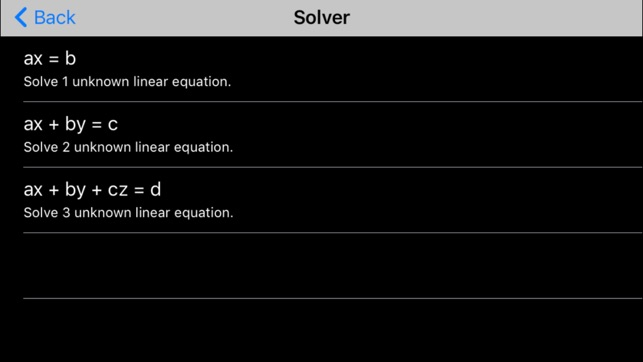•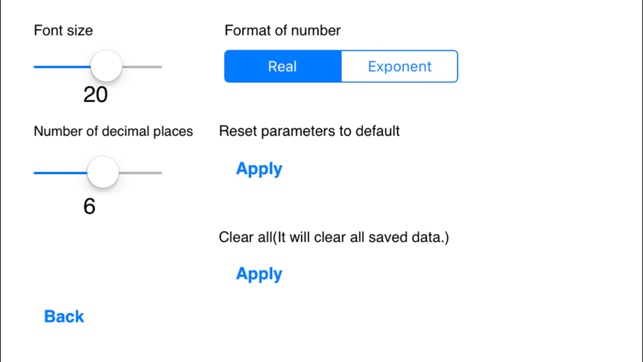## Description

1. This calculator can be calculated by inputting the formula unlike a usual calculator.
2. The variable can be used in the formula. You can use alphabet(a-z) and greek alphabet(α-ω) as the variable. If this function is used, for example the following equations will be created,

a*x*y + b*y + c*z + 10

you can perform changing and re-calculating the value of a variable later simply.

3. You can edit formula with moving caret.
4. You can create the simple graph(the division by zero is not supported) by using the created formula.
5. You can send the created formula with mail.
6. You can solve simultaneous equation like the followings,

case1:Solve 1 unknown linear equation.
a*x = b

case2:Solve 2 unknown linear equation.
a1*x + b1*y = c1
a2*x + b2*y = c2

case3:Solve 3 unknown linear equation.
a1*x + b1*y + c1*z =d1
a2*x + b2*y + c2*z =d2
a3*x + b3*y + c3*z =d3

## What’s New

Version 2.0.7

All abs have been deleted.

## Ratings and Reviews

3.4 out of 5
5 Ratings

5 Ratings

Pkup10 ,

### Kind of clunky and unintuitive...

FormulaCalc+ is designed for the iPhone an is very limited in features. It includes a basic scientific calculator with trigonometric functions but is not very intuitive or user friendly. One example is that the after pressing equal when you begin typing the next set of numbers the prior calculation isn't cleared. It has a very basic graphing feature that doesn't do much more than just viewing a function.

## Information

Seller
Malloc Code Corporation
Size
4.5 MB
Category
Education
Compatibility

Requires iOS 8.0 or later. Compatible with iPhone, iPad, and iPod touch.

Languages

English, Japanese

Age Rating
Rated 4+
Price
\$0.99

## Supports

•